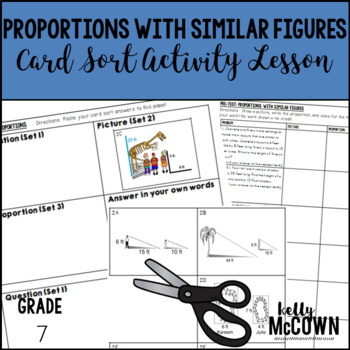## Monday, October 24, 2016

### Teaching Proportions & Percents {Grade 7}

One skills that is often difficult for seventh grade students is learning proportions and percents. In sixth grade students learn the foundations for how to solve proportions for seventh grade. Now that they are in seventh grade they need to use those foundational skills to build upon. Students often make mistakes with setting up proportions, multiplying and solving proportions, and setting up to solve percents.

I have listed here activities to review and practice Proportions and Percents. I hope these help jump start your lesson planning into the world of Proportional Relationships. The key to getting your students involved in the learning process is to include interactive, visual, engaging activities for them to actively participate in.These interactive notebook activities are intended to help students understand how to use proportional relationships to solve multistep ratio and percent problems, understand that rewriting an expression in different forms in a problem context can shed light on the problem, and solve multi-step real-life and mathematical problems posed with rational numbers.

Included are:
-4 different Proportions & Percents activities to engage students
-Teacher facilitated activity for 60-90 minutes of classroom time
-Activities include: Vocabulary, finding the part, whole, or Percent, Finding Sales tax and Discounts, and Finding Simple Interest.
-Examples of Completed Interactive Math Notebook Activities are includedThis math station activity is intended to help students understand how to use proportional relationships to solve multistep ratio and percent problems, understand that rewriting an expression in different forms in a problem context can shed light on the problem, and solve multi-step real-life and mathematical problems posed with rational numbers.

Included are:
-6 different stations to engage students
-Teacher facilitated activity for 60-90 minutes of classroom time
-Stations include:
1. Vocabulary
3. Practice of Proportions & Percents
4. Additional Practice of Proportions & Percents
5. Real World applications of Proportions & Percents
6. Word problems of Proportions & Percents
-Student Station Guide helps students record their answersThese math foldable notes are for students to be able to understand compute unit rates, ratios with fractions, measure quantities in like or different units, recognize and represent proportional relationships, decide whether two quantities are proportional, identify the constant of proportionality (unit rate), show the unit rate in tables, graphs and equations, represent proportional relationships by equations, explain points on a graph of a proportional relationship, and use proportional relationships to solve multistep ratio and percent problems.

Included are:
-7 different NO PREP Math Foldable Notes to engage students in note taking
-Teacher facilitated activity for 15-20 minutes of classroom time each
-PRINT & GO foldable notes that are ready with NO PREP
-Directions for using Math Foldables in your classroom
-Options for scaffolding with blank templates
-Foldables included:
Foldable: Percent of a Number
Foldable: Percent Proportion
Foldable: Percent and Estimation
Foldable: The Percent Equation
Foldable: Percent of Change
Foldable: Sales Tax and Discount
Foldable: Simple Interest
Foldable: Four Blank TemplatesThis lesson unit is intended to help you assess how well students are able to identify when two quantities vary in direct proportion to each other, represent two quantities as directly proportional relationships, and solve proportional problems using efficient methods.Included in this lesson are:
-card sort activity with concept development of proportions
-one extension activity for students to deepen their conception of proportions
-answer keys for all the assessments and activities
-how the lesson is tied to common core and mathematical practicesThis November Math NO PREP packet that will keep your seventh graders engaged! This packet is just plain fun. Not only is it PACKED with seventh-grade common core math problems, it also gives students fun coloring, puzzles, and problem solving. Use this packet for bellwork, classwork, extra credit, fast finishers, or homework!
Topics Covered: -Rational Numbers
-Rates and Proportionality
-Graphing on a Coordinate Plane
-Proportions
-Percents

I hope these resources are helpful in getting your students engaged in Proportions & Percents. Keeping students actively engaged in the learning process will ensure that they are in charge of their own learning. The goal of learning Proportions is to set up the foundations for Algebra skills in the future. Students must master proportions in seventh grade to be able to achieve success in Algebra. Your teaching is important and the students need to actively be engaged in the process.